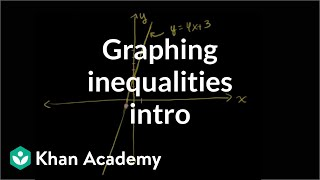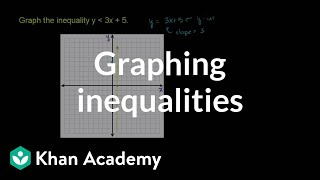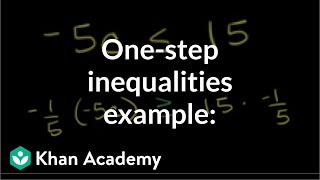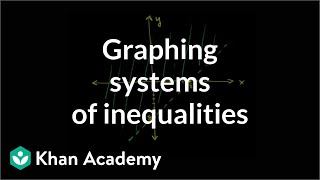# Testing solutions of inequalities

##By Khan Academy

A solution to an inequality makes that inequality true. Learn how to test if a certain value of a variable makes an inequality true.# Constraint solution sets of systems of linear inequalities

##By Khan Academy

Given the graph of a system of inequalities, Sal finds the x-values that make the ordered pair (x,-2) a solution of the system, which is the solution set constrained to y=-2. Then he solves a similar problem where x is constrained to 4.# Constraint solution sets of two-variable linear inequalities

##By Khan Academy

Sal determines which x-values make the ordered pair (x,-7) a solution of 2x-7y<25. He also solves a similar problem where the inequality is given as a graph.# Check solutions of two-variable linear inequalities

##By Khan Academy

Sal determines whether the ordered pairs (3,5) and (1,-7) are solutions of the inequality 5x-3yï¿½ï¿½ï¿½ï¿½ï¿½ï¿½ï¿½ï¿½ï¿½ï¿½ï¿½ï¿½ï¿½ï¿½ï¿½ï¿½ï¿½ï¿½ï¿½ï¿½ï¿½25. He discussed the problem both algebraically and graphically.##By Khan Academy

Watch this explanation of how to solve 1-step inequalities and respresent the solution on a number line. Solving an inequality means finding all of its solutions. A solution of an inequality is a number which when substituted for the variable makes the inequality a true statement. Unlike an equation an inequality has many solutions.# Multi-step linear inequalities

##By Khan Academy

Sal solves the inequality -3p-7# Graph inequalities and check solutions

##By Khan Academy

Learn how to graph two-variable linear inequalities.# Graph inequalities and check solutions

##By Khan Academy

Learn how to graph two-variable linear inequalities.# Graph inequalities and check solutions

##By Khan Academy

Sal graphs the inequality y<3x+5.# Check solutions of systems of inequalities

##By Khan Academy

Sal checks whether the ordered pair (2,5) is a solution of the following system: yï¿½ï¿½ï¿½ï¿½ï¿½ï¿½ï¿½ï¿½ï¿½ï¿½ï¿½ï¿½ï¿½ï¿½ï¿½ï¿½ï¿½ï¿½ï¿½ï¿½ï¿½2x+1 and x>1.# Graph inequalities and check solutions

##By Khan Academy

Sal graphs the inequality y<3x+5.# Two-variable linear inequalities word problems

##By Khan Academy

Sal is given a two-variable linear inequality that models a context about watering plants, and answers a question about the solution set of the inequality in terms of the context.# Two-variable linear inequalities word problems

##By Khan Academy

Sal is given a two-variable linear inequality that models a context about watering plants, and answers a question about the solution set of the inequality in terms of the context.# One-step inequalities

##By Khan Academy

In addition to solving the inequality, we'll graph the solution. Remember to swap if you mutiply both sides of the inequality by a negative number.# Two-variable linear inequalities word problems

##By Khan Academy

Sal is given a two-variable linear inequality that models a context about watering plants, and answers a question about the solution set of the inequality in terms of the context.# Two-variable linear inequalities word problems

##By Khan Academy

Sal is given a two-variable linear inequality that models a context about watering plants, and answers a question about the solution set of the inequality in terms of the context.# Multi-step linear inequalities

##By Khan Academy

Sal solves the inequality 5x+7>3(x+1), draws the solution on a number line and checks a few values to verify the solution.# Graph systems of inequalities and check solutions

##By Khan Academy

Sal determines the solution set of the following system: yï¿½ï¿½ï¿½ï¿½ï¿½ï¿½ï¿½ï¿½ï¿½ï¿½ï¿½ï¿½ï¿½ï¿½ï¿½ï¿½ï¿½ï¿½ï¿½ï¿½ï¿½2x+1 and y<2x-5 and x>1. He does that by graphing the system and analyzing the graph.# Graph systems of inequalities and check solutions

##By Khan Academy

Learn how to graph systems of two-variable linear inequalities.# Graph systems of inequalities and check solutions

##By Khan Academy

Sal determines the solution set of the following system: yï¿½ï¿½ï¿½ï¿½ï¿½ï¿½ï¿½ï¿½ï¿½ï¿½ï¿½ï¿½ï¿½ï¿½ï¿½ï¿½ï¿½ï¿½ï¿½ï¿½ï¿½2x+1 and y<2x-5 and x>1. He does that by graphing the system and analyzing the graph.